Technical Article

# Negative Feedback, Part 6: New and Improved Stability Analysis

November 27, 2015 by Robert Keim

## This article will show you a handy alternative approach to assessing stability via open-loop gain and the feedback factor.

This article will show you a handy alternative approach to assessing stability via open-loop gain and the feedback factor.

### Supporting Information

Just so you don’t have to switch pages every time you want to ponder the general feedback structure, here is the diagram presented in the first article: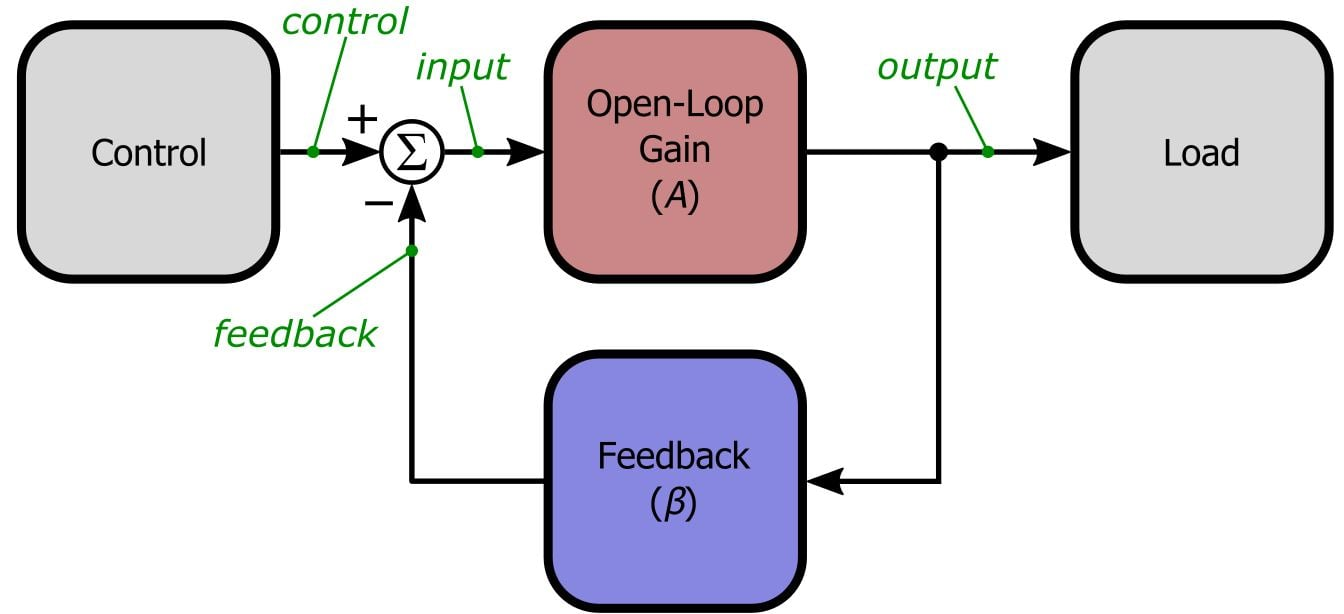And here is the LTSpice simulation circuit discussed in the previous installment: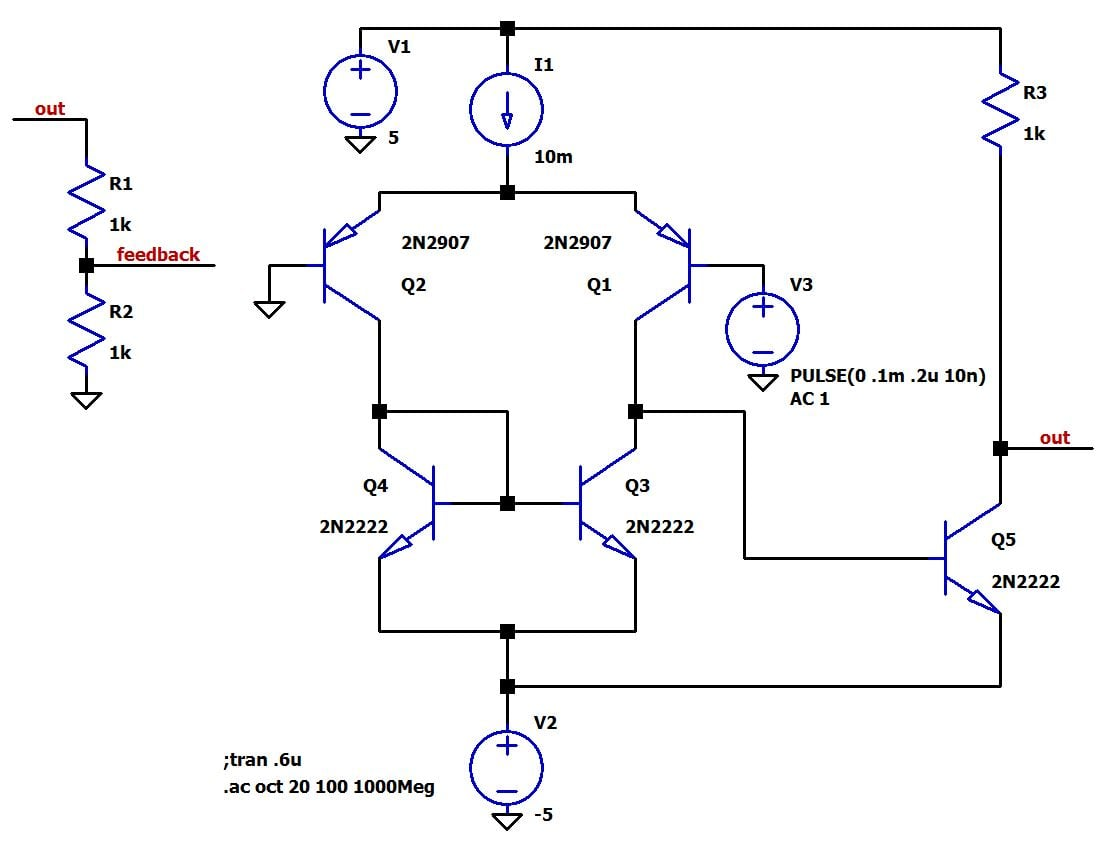We will use this same circuit for the simulations in this article.

### Separating A from β

We now know that by plotting the gain and phase shift of a negative feedback amplifier’s loop gain—denoted by , where A is always a function of frequency and β can be considered a function of frequency if necessary—we can determine two things: 1) whether the amplifier is stable, and 2) whether the amplifier is sufficiently stable (rather than marginally stable). The first determination is based on the stability criterion, which states that the magnitude of the loop gain must be less than unity at the frequency where the phase shift of the loop gain is 180°. The second is based on the amount of gain margin or phase margin; a rule of thumb is that the phase margin should be at least 45°.

It turns out that we can effectively analyze stability using an alternative and somewhat simplified approach in which open-loop gain A and feedback factor β are depicted as separate curves on the same axes. Consider the following plot for the discrete BJT amplifier with a frequency-independent (i.e., resistor-only) feedback network configured for β = 0.5: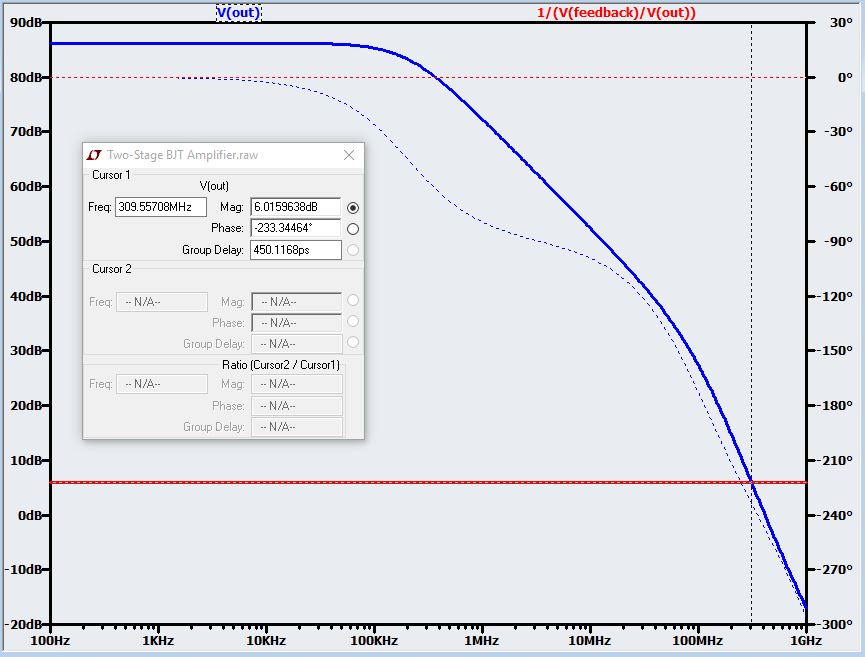Here you see V(out), which corresponds to the open-loop gain, and 1/(V(feedback)/V(out)). If you recall that β is the percentage (expressed as a decimal) of the output fed back and subtracted from the input, you will surely recognize that this second trace is simply 1/β. So why did we plot 1/β? Well, we know that loop gain is A multiplied by β, but in this plot the y-axis is in decibels and is thus logarithmic. Our high school math teachers taught us that multiplication of ordinary numbers corresponds to addition with logarithmic values, and likewise numerical division corresponds to logarithmic subtraction. Thus, a logarithmic plot of A multiplied by β can be represented as the logarithmic plot of A plus the logarithmic plot of β. Remember, though, that the above plot includes not β but rather 1/β, which is the equivalent of negative β on a logarithmic scale. Let’s use some numbers to clarify this:

$\beta=0.5\ \Rightarrow\ 20\log\left(\beta\right)\approx-6\ dB$

$\frac{1}{\beta}=2\ \Rightarrow\ 20\log\left(\frac{1}{\beta}\right)\approx6\ dB$

Thus, in this logarithmic plot, we have 20log(A) and -20log(β), which means that to reconstruct 20log() we need to subtract the 1/β curve from the A curve:

$20\log\left(A\beta\right)=20\log\left(A\right)+20\log\left(\beta\right)\ \Rightarrow\ 20\log\left(A\beta\right)=20\log\left(A\right)-\left(-20\log\left(\beta\right)\right)$

$\Rightarrow\ 20\log\left(A\beta\right)=20\log\left(A\right)-20\log\left(\frac{1}{\beta}\right)$

### Stability Criterion Revisited

The first implication of the foregoing discussion is that reaches unity gain at the frequency where the two traces intersect:

$20\log\left(A\beta\right)=20\log\left(A\right)-20\log\left(\frac{1}{\beta}\right)\ \Rightarrow\ if\ \ 20\log\left(A\right)=20\log\left(\frac{1}{\beta}\right),\ 20\log\left(A\beta\right)=0$

Thus, we can reformulate the theoretical stability criterion as follows: if the phase shift is less than 180° at the frequency where the logarithmic magnitudes of A and 1/β intersect, the amplifier is stable.

In case you haven’t realized this yet, the 20log(1/β) trace also conveys the closed-loop gain characteristics—as we saw so many articles ago, closed-loop gain is very closely approximated as 1/β. This is handy, because with this one plot we can easily assess stability in relation to closed-loop gain. Here is a magnified view of the intersection region in the previous plot: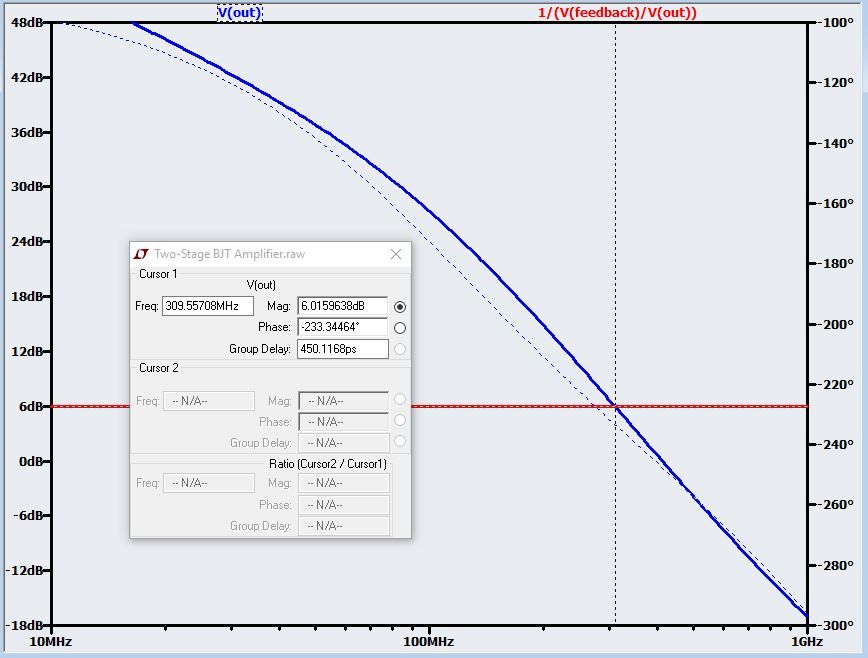The phase shift at the intersection point is 233°, which means that this amplifier is wildly unstable with a closed-loop gain of 6 dB. By simply sliding the 20log(1/β) curve up or down, we can readily determine what sort of stability we will get with different closed-loop gain configurations. In the previous article we found that this amplifier needs a closed-loop gain of about 78 V/V (or 38 dB) to be sufficiently stable; if we change the resistive divider to obtain β = 0.013, we get the following: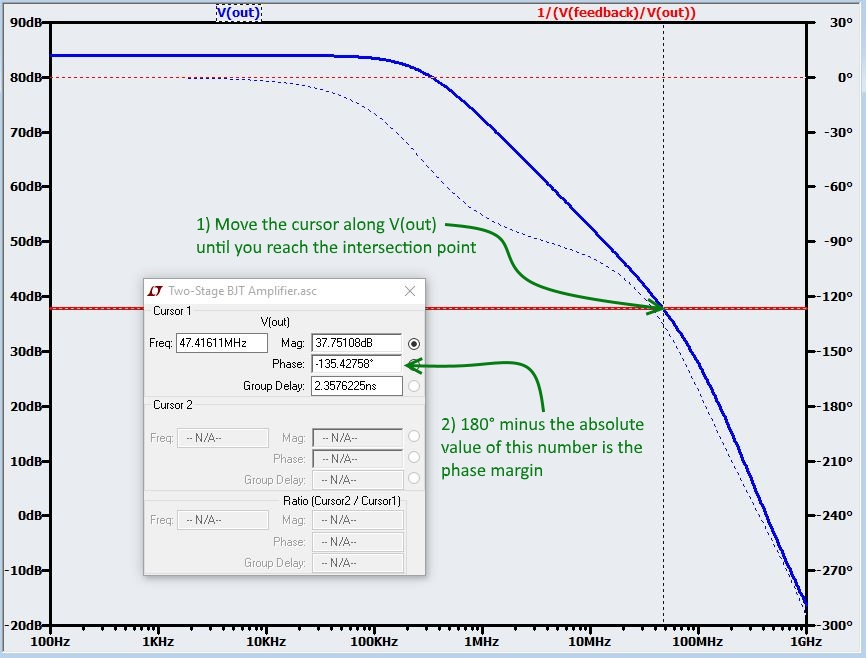As you can see, this alternative method confirms that a closed-loop gain of 38 dB provides a phase margin of 45°.

### Simplify: Slope Instead of Margin

Now we come to the technique that really distinguishes this alternative method from the standard loop-gain method presented in the previous article. First we need to think about phase shift and how it relates to magnitude response:

1. Each pole in a circuit’s transfer function contributes 90° of phase shift.
2. The phase shift becomes significant approximately one frequency decade before the pole frequency and approaches 90° approximately one frequency decade after the pole frequency; the pole frequency itself sees a phase shift of 45°.
3. Each pole causes the magnitude response to decrease at a rate of 20 dB per frequency decade. Thus, a one-pole response has a constant roll-off of 20 dB/decade. A second pole brings an additional 20 dB/decade, such that beyond the frequency of the second pole, the roll-off is 40 dB/decade.
4. The theoretical phase shift at the second pole frequency is 135° (90° from the first pole plus the additional 45° seen by the pole frequency itself).
5. The second pole frequency is the point at which the magnitude of the roll-off transitions from 20 dB/decade to 40 dB/decade.Therefore, taking into account these five points, we can state the following: an amplifier with a frequency-independent feedback network will be sufficiently stable if the 20log(1/β) magnitude intersects the 20log(A) magnitude in the portion of the 20log(A) magnitude where roll-off is 20 dB/decade. Why sufficiently stable rather than merely theoretically stable? Because the frequency of the second pole has a phase shift of approximately 135°, and thus an intersection before this pole indicates that the phase shift at the loop gain’s unity-gain frequency is at least 45° less than 180° (180° – 135° = 45°). In other words, an intersection in the 20 dB/decade roll-off portion indicates that our phase margin satisfies the 45° rule of thumb.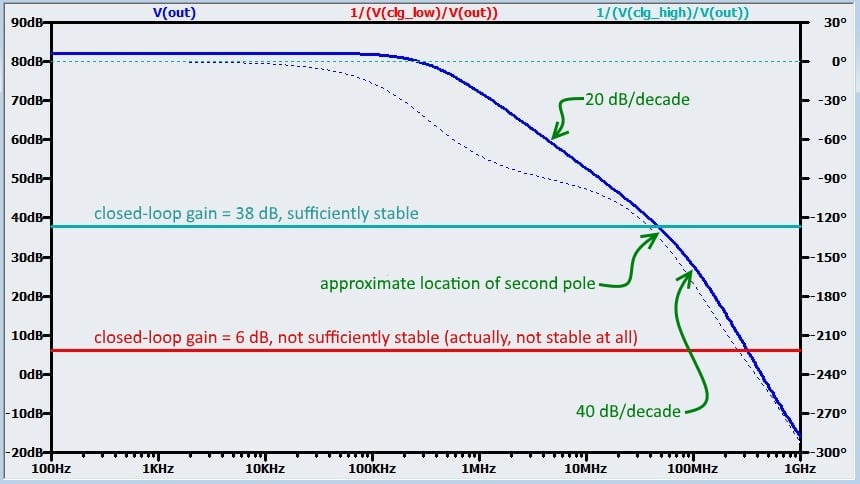### Conclusion

This result regarding the roll-off slope at the intersection point is highly exhilarating, but it gets even better. We can actually generalize this technique to include frequency-dependent feedback networks, as follows: if the difference between the slope of the open-loop gain’s magnitude response and the slope of the feedback network’s magnitude response is not greater than 20 dB/decade at the point of intersection, the amplifier is sufficiently stable. The alternative stability-analysis method discussed in this article is somewhat simpler than the standard method, and it is more practical in situations when you have no ability or desire to perform simulations—you can get a good idea of the circuit’s stability for different closed-loop gains with nothing more than approximate pole locations or a datasheet-style open-loop gain plot. But as you will see in the next article, this method can be especially helpful when you are intuitively or analytically investigating the stability of negative-feedback circuits in which the frequency response of the feedback network must be taken into consideration.

Next Article in Series: Negative Feedback, Part 7: Frequency-Dependent Feedback•RK37 May 20, 2020